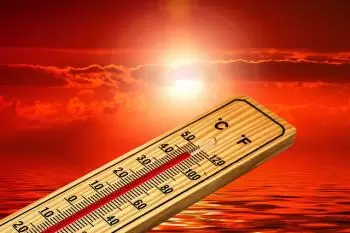# What Is the Difference Between Heat and Temperature?

Heat and temperature are two concepts that are so closely related that they are often confused with each other. The differences between heat and temperature are:1. Heat is the thermal energy transferred between two or more bodies, while the temperature is a thermodynamic property that measures the thermal state of a body.

2. Heat is measured in Joules (J) or calories (cal), while the temperature is measured in Kelvin, degrees Celsius, or degrees Fahrenheit.

3. The calorimeter is the device that measures energy in transfer, that is, heat, while we can use a temperature sensor or a thermometer to measure the temperature.

4. The effect of heat depends on the amount of matter there is, not the temperature.

## What Is Called Heat?

Heat is a form of thermal energy that is transferred between two bodies that are at different temperatures.

According to the International Energy System, the unit to measure heat is the joule (J). However, in some fields, the unit calorie (cal) is used.

Heat transfer can be done by radiation, convection, and translation.

## What Is Temperature?

Temperature, on the other hand, is a property that indicates the thermal state of a body, that is, the total kinetic energy of the molecules that make up the body itself. It is the degree of hotness or coldness of an object.

When heat is transferred to a body, its molecular motion increases: this phenomenon gives rise to kinetic energy (the energy of motion). Temperature is, therefore, the measure of the average kinetic energy of the particles that make up a body.

The temperature does not depend on the number of particles in a body. It depends on the molecule's average speed that composes it. If a body has a higher temperature, it means that its molecules move faster.

The temperature's SI unit is Kelvin (K). Nevertheless, we can also use degrees Celsius (° C) or degrees Fahrenheit (ºF).

## What Is the Relationship Between Heat and Temperature?

According to the first law of thermodynamics, if two bodies at different temperatures are in contact, there is an energy exchange in the form of heat.

Under normal conditions, if we remove heat from a body, the temperature drops. On the other hand, if we supply heat, the temperature increases.

However, this is not the case when there is a phase change. For example, the heat added during a phase change from solid to liquid does not imply an increase in temperature.

Specific heat capacity is the amount of heat to be supplied to (or taken out of) the unit mass of a system to increase (or decrease) its temperature by one degree in a thermodynamic process.

Author:

Published: June 7, 2021
Last review: June 7, 2021